### Computer Memory

From the point of view of a running program, memory can be understood as a long tape of bytes, and the program can read from or write to certain places on the tape. There are two concepts that we need to know:

• address, an integer that specifies a place on the tape
• data, the byte stored in each place on the tape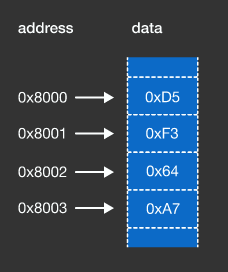We often use hexadecimal for data. The beginning address of the tape (or memory) is determined by the OS. For this example, the byte at the address 0x8000 contains the data 0xD5. In modern computer architectures, one memory address points to one byte. Therefore, the address of the next byte is 0x8001, which contains the data 0xF3.

Programs access memory by running CPU instructions and specifying the target address. For example:

• read 1 byte from address 0x8000
• write 2 bytes to address 0x8002 (this means 0x8002 and 0x8003)

# Linear Memory Model

When working with memory, we often use sizes that are $2 ^ N$ for convention. We use units like MiB ($2 ^ {20} = 1024 \times 1024$ bytes) or GiB ($2 ^ {30} = 1024 \times 1024 \times 1024$ bytes), instead of the regular units like MB ($10 ^ 6$ bytes) or GB ($10 ^ 9$ bytes).

Given a computer that has $1 \text{ MiB}$ of memory, where the first address of the memory is determined by the OS to be 0xC00000, what is the address of the last byte of memory?

# Linear Memory Model

Since one byte can hold values no larger than 255, we often work with integers that span multiple bytes to hold larger numbers. For example, we need at least 2 bytes of memory to represent the number 43690, which is 0xAAAA in hexadecimal.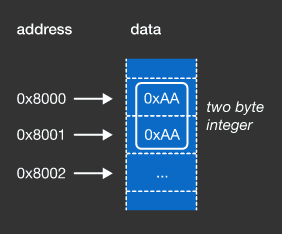At least how many bytes of memory do we need to represent the decimal number 1,000,000,000?

# Linear Memory Model

Since each byte has a memory address, it’s worth asking: in what order do we store the bytes that compose a multi-byte integer? Do we store the least-significant (rightmost) byte in the first memory address, or do we store the most-significant (leftmost) byte in the first memory address? The answer is that it depends on the endianness of your computer’s architecture. Storing the most-significant byte first is called big-endian. Storing the least-significant byte first is called little-endian.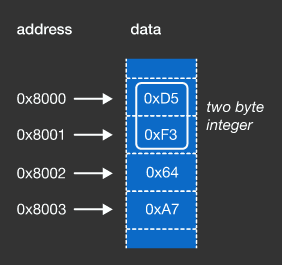Given a little-endian computer architecture, and memory that looks like the above diagram, the address 0x8000 and 0x8001 together are used to describe a 2-byte integer. What is the integer in decimal (base 10)?

# Linear Memory Model

The entire memory can be considered as a giant 1-dimensional tape of bytes. However, to make it easy to work with, we’ll often wrap it across multiple lines such that there are many bytes per line (often 16), and the memory address of the first byte on each line will be specified in a column to the left of the resulting table. To get the memory address of an arbitrary byte, look up the memory address of the first byte on that line, count the number of bytes to the left of the target byte, and add that number to the memory address of the first byte.

This method of displaying memory is called a hex dump and is quite common in debuggers, packet sniffers, hex editors and the like.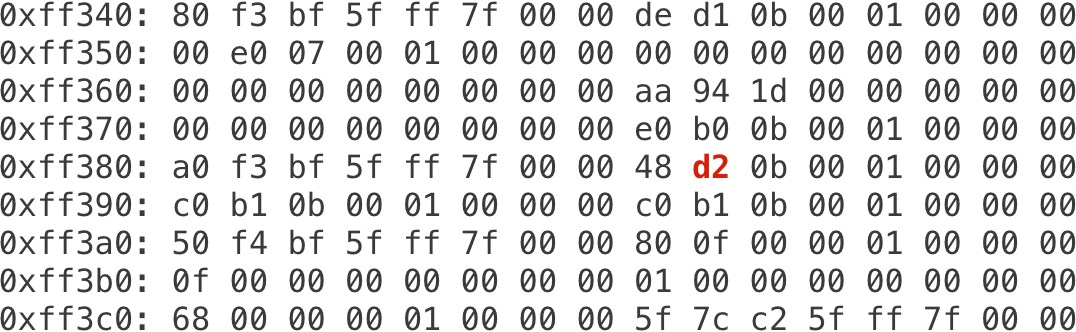What is the memory address of the red bolded byte?

# Linear Memory Model

The x86-64 architecture is little-endian. Below is a memory dump from a computer running on this architecture: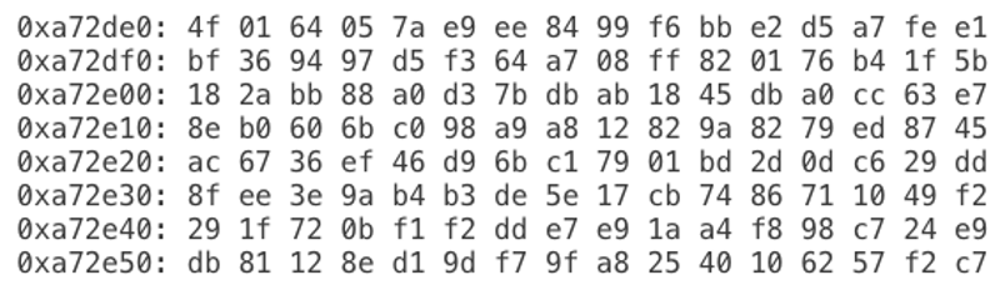What is the value of the 32-bit integer stored at memory address 0xa72e29? Answer in decimal (base 10).

×